6226.0 - Participation, Job Search and Mobility, Australia, February 2015
ARCHIVED ISSUE Released at 11:30 AM (CANBERRA TIME) 21/06/2016  First Issue
Page tools:Print All

TECHNICAL NOTE DATA QUALITY

INTRODUCTION

1 Since the estimates in this publication are based on information obtained from occupants of a sample of households, they are subject to sampling variability. That is, they may differ from those estimates that would have been produced if all households had been included in the survey or a different sample was selected. One measure of the likely difference is given by the standard error (SE), which indicates the extent to which an estimate might have varied by chance because only a sample of households was included. There are about two chances in three (67%) that a sample estimate will differ by less than one SE from the number that would have been obtained if all households had been included, and about 19 chances in 20 (95%) that the difference will be less than two SEs.

2 Another measure of the likely difference is the relative standard error (RSE), which is obtained by expressing the SE as a percentage of the estimate.
RSE% = (SE/estimate ) x 100

3 RSEs for Participation, Job Search and Mobility estimates have been calculated using the Jackknife method of variance estimation. This process involves the calculation of 30 'replicate' estimates based on 30 different sub-samples of the original sample. The variability of estimates obtained from these sub-samples is used to estimate the sample variability surrounding the main estimate.

4
The Excel spreadsheets in the Downloads tab contain all the tables produced for this release and the calculated RSEs for each of the estimates. The RSEs for estimates have been calculated using the Jackknife method.

5
In the tables in this publication, only estimates (numbers, percentages and means) with RSEs less than 25% are considered sufficiently reliable for most purposes. However, estimates with larger RSEs have been included. Estimates with an RSE in the range 25% to 50% should be used with caution while estimates with RSEs greater than 50% are considered too unreliable for general use. All cells in the Excel spreadsheets with RSEs greater than 25% contain a comment indicating the size of the RSE. These cells can be identified by a red indicator in the corner of the cell. The comment appears when the mouse pointer hovers over the cell.

CALCULATION OF STANDARD ERROR

6
RSEs are routinely presented as the measure of sampling error in this publication and related products. SEs can be calculated using the estimates (counts or means) and the corresponding RSEs.

7
An example of the calculation of the SE from an RSE follows. Datacube 31 shows that the estimated number of person who have worked for less than 12 months was 2,145,500 in February 2015, and the RSE for this estimate was 1.7%. The SE is:
SE of estimate
= (RSE / 100) x estimate
= 0.017 x 2,145,500
= 36,500 (rounded to the nearest 100)

8 Therefore, there are about two chances in three that the value that would have been produced if all households had been included in the survey would fall within the range 2,109,000 to 2,182,000 and about 19 chances in 20 that the value would fall within the range 2,072,500 to 2,218,500. This example is illustrated in the following diagram.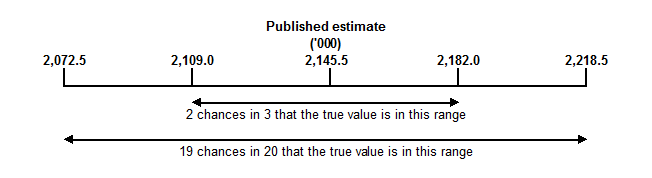PROPORTIONS AND PERCENTAGES

9
Proportions and percentages formed from the ratio of two estimates are also subject to sampling errors. The size of the error depends on the accuracy of both the numerator and the denominator. A formula to approximate the RSEs of proportions not provided in the spreadsheets is given below. This formula is only valid when x is a subset of y.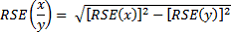10 Considering the Data Cube 31, of the 2,145,500 persons who worked less than 12 months with current employer/business, 604,000 or (28.2%) worked 3 months or less. The RSE of 604,000 is 3.1% and the RSE for 2,145,500 is 1.7%. Applying the above formula, the RSE for the proportion of persons who worked 3 months or less: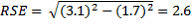11
Therefore, the SE for the proportion of persons who worked 3 months or less was 0.7 percentage points (= (28.2/100) x 2.6). Therefore, there are about two chances in three that the proportion of persons who worked less than 12 months and how also worked 3 months or less is between 27.5% and 28.9%, and 19 chances in 20 that the proportion was within the range 26.8% to 29.6%.

SUMS OR DIFFERENCES BETWEEN ESTIMATES

12
Published estimates may also be used to calculate the sum of two or more estimates, or the difference between two survey estimates (of numbers, means or percentages) where these are not provided in the spreadsheets. Such estimates are also subject to sampling error.

13
The sampling error of the difference between two estimates depends on their SEs and the relationship (correlation) between them. An approximate SE of the difference between two estimates (x–y) may be calculated by the following formula: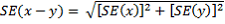14
The sampling error of the sum of two estimates is calculated in a similar way. An approximate SE of the sum of two estimates (x+y) may be calculated by the following formula: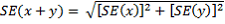15 Considering the example in paragraph 7, the estimated number of person who have worked for less than 12 months was 2,145,500, and the SE for this estimate was 36,500. From the Data Cube 31, the estimate of persons who have worked for 1—2 years was 2,350,400 and the SE was 28,200. The estimate of persons who worked for less than 3 years is:
2,145,500 + 2,350,400 = 4,495,900

16 The SE of the estimate of persons who have worked for less than 3 years is: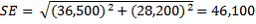17
Therefore, there are about two chances in three that the value that would have been produced if all households had been included in the survey would fall within the range 4,431,200 to 4,560,600 and about 19 chances in 20 that the value would fall within the range 4,366,500 to 4,625,300.

18
While these formulae will only be exact for sums of, or differences between, separate and uncorrelated characteristics or subpopulations, it is expected to provide a good approximation for all sums or differences likely to be of interest in this publication.

STANDARD ERRORS OF MEANS AND SUMS

19
The estimates of means and sums of continuous variables are subject to sampling variability and random adjustment. As for population estimates, the variability due to sampling and random adjustment is combined into the calculated Standard Error, and the Relative Standard Error is reported. The component of variability arising from sampling is calculated using the Jackknife method.

SELECTED ESTIMATES AND RSES

The following table shows how the RSEs are displayed for selected estimates in the datacubes.

 T1 – Employed Persons who started their current job in the previous 12 months, Age group years and state or territory of usual residence – By sexSex of personMales Females Persons Weighted CountAge group (years)15–19 '000 158.3 160.9 319.220–24 '000 206.9 188.2 394.125–34 '000 344.4 286.0 629.435–44 '000 199.1 175.4 375.845–54 '000 144.4 130.8 273.954–59 '000 54.2 33.8 89.660–64 '000 27.0 18.6 45.265–69 '000 8.6 *5.6 14.770 and over '000 *4.1 **2.1 8.2 State or territory of usual residenceNew South Wales '000 345.8 292.3 639.3Victoria '000 292.0 249.7 539.5Queensland '000 236.1 228.2 465.9South Australia '000 71.2 58.7 129.6Western Australia '000 152.1 121.3 270.7Tasmania '000 17.9 16.8 35.2Northern Territory '000 16.7 14.0 30.8Australian Capital Territory '000 19.4 18.6 37.8 Total '000 1144.0 1002.0 2145.5 Relative Standard Error of weighted countAge group (years)15–19 RSE % 6.3 5.4 4.520–24 RSE % 4.2 5.8 4.025–34 RSE % 3.7 3.8 2.935–44 RSE % 5.3 5.0 4.045–54 RSE % 7.3 5.8 4.854–59 RSE % 11.5 12.2 8.260–64 RSE % 12.5 14.9 9.665–69 RSE % 21.9 35.6 18.370 and over RSE % 36.8 57.4 23.8 State or territory of usual residenceNew South Wales RSE % 3.2 4.0 2.9Victoria RSE % 4.2 3.6 3.0Queensland RSE % 5.1 4.9 3.9South Australia RSE % 5.2 6.8 4.0Western Australia RSE % 5.7 5.5 3.8Tasmania RSE % 8.9 10.6 7.0Northern Territory RSE % 9.5 7.8 6.8Australian Capital Territory RSE % 11.3 8.9 7.5 Total RSE % 2.2 2.0 1.7 * estimate has a relative standard error of 25% to 50% and should be used with caution ** estimate has a relative standard error greater than 50% and is considered too unreliable for general use

SIGNIFICANCE TESTING

21
A statistical test for any comparisons between estimates can be performed to determine whether it is likely that there is a significant difference between two corresponding population characteristics. The standard error of the difference between two corresponding estimates (x and y) can be calculated using the formula in paragraph 9. This standard error is then used to calculate the following test statistic: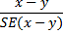22
If the value of this test statistic is greater than 1.96 then there is evidence, with a 95% level of confidence, of a statistically significant difference in the two populations with respect to that characteristic. Otherwise, it cannot be stated with confidence that there is a difference between the populations with respect to that characteristic.

23
The imprecision due to sampling variability, which is measured by the SE, should not be confused with inaccuracies that may occur because of imperfections in reporting by respondents and recording by interviewers, and errors made in coding and processing data. Inaccuracies of this kind are referred to as non-sampling error, and they occur in any enumeration, whether it be a full count or sample. Every effort is made to reduce non-sampling error to a minimum by careful design of questionnaires, intensive training and supervision of interviewers, and efficient operating procedures.